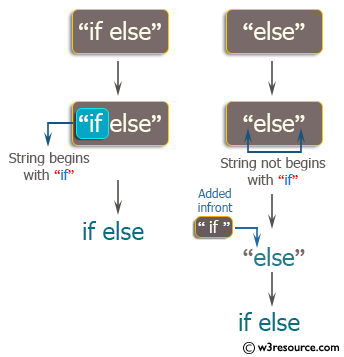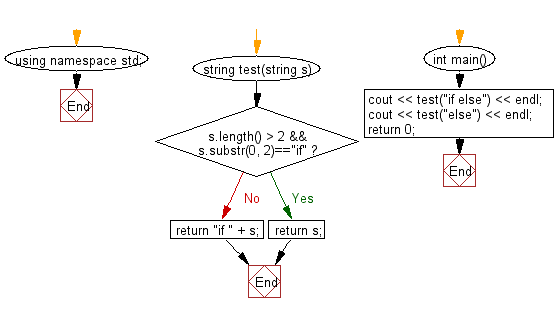﻿ C++ : New string where if is added to the front of a string# C++ Exercises: Create a new string where 'if' is added to the front of a given string

## C++ Basic Algorithm: Exercise-5 with Solution

Write a C++ program to create a new string where 'if' is added to the front of a given string. If the string already begins with 'if', return the string unchanged.

Sample Solution:

C++ Code :

``````#include <iostream>

using namespace std;

string test(string s)
{
if (s.length() > 2 && s.substr(0, 2)=="if")
{
return s;
}
return "if " + s;
}

int main()
{
cout << test("if else") << endl;
cout << test("else") << endl;
return 0;
}
``````

Sample Output:

```if else
if else
```

Pictorial Presentation:Flowchart:C++ Code Editor: